# Newtons First Law Reviewed Newtons First Law An

• Slides: 20Newton’s First Law Reviewed Newton’s First Law: An object at rest or an object in motion at constant speed will remain at rest or at constant speed in the absence of a resultant force. A glass is placed on a board and the board is jerked quickly to the right. The glass tends to remain at rest while the board is removed.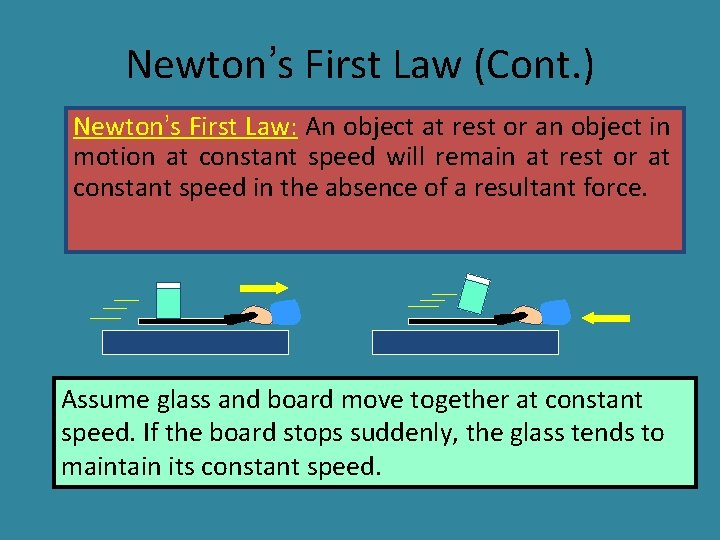Newton’s First Law (Cont. ) Newton’s First Law: An object at rest or an object in motion at constant speed will remain at rest or at constant speed in the absence of a resultant force. Assume glass and board move together at constant speed. If the board stops suddenly, the glass tends to maintain its constant speed.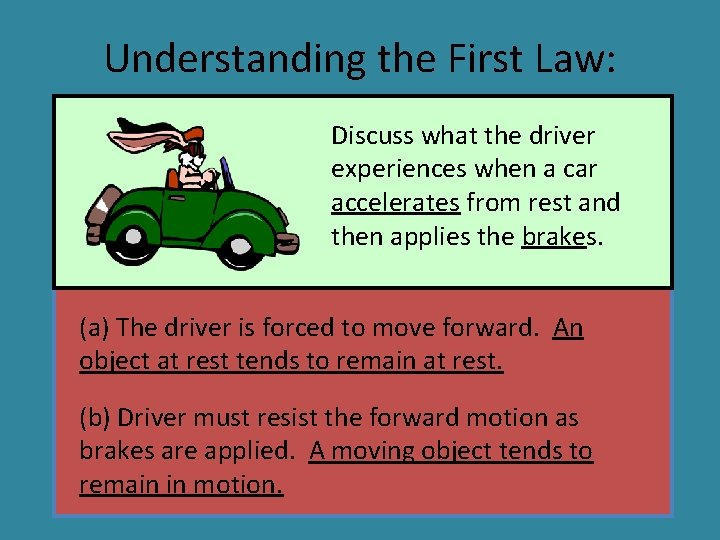Understanding the First Law: Discuss what the driver experiences when a car accelerates from rest and then applies the brakes. (a) The driver is forced to move forward. An object at rest tends to remain at rest. (b) Driver must resist the forward motion as brakes are applied. A moving object tends to remain in motion.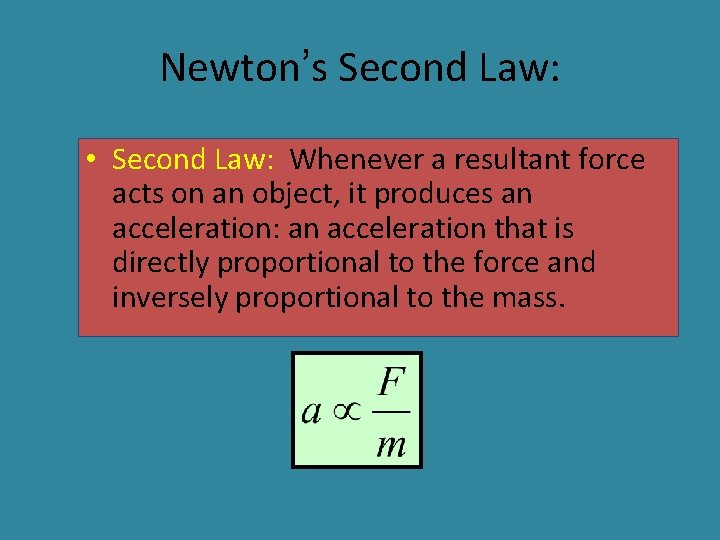Newton’s Second Law: • Second Law: Whenever a resultant force acts on an object, it produces an acceleration: an acceleration that is directly proportional to the force and inversely proportional to the mass.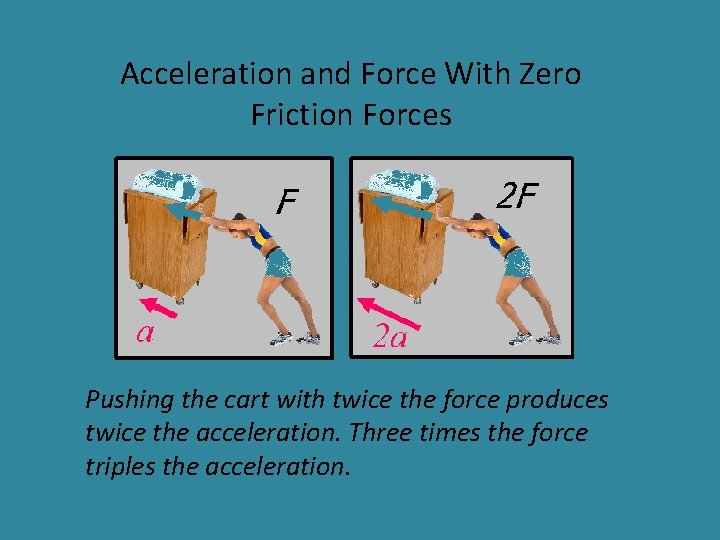Acceleration and Force With Zero Friction Forces Pushing the cart with twice the force produces twice the acceleration. Three times the force triples the acceleration.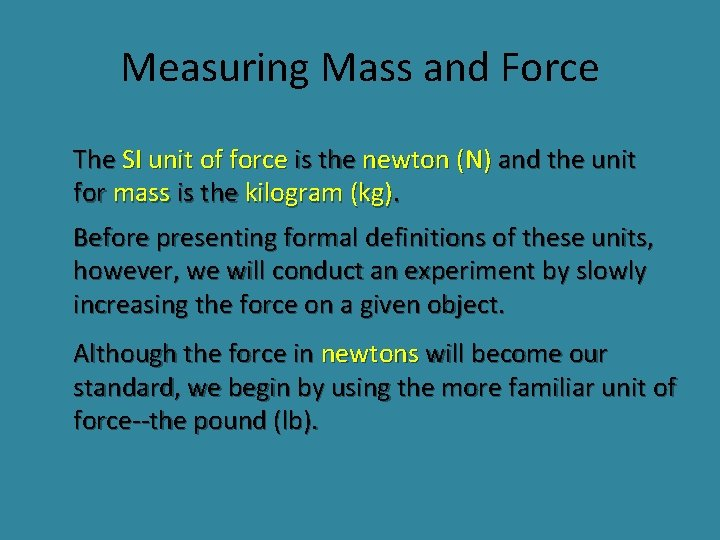Measuring Mass and Force The SI unit of force is the newton (N) and the unit for mass is the kilogram (kg). Before presenting formal definitions of these units, however, we will conduct an experiment by slowly increasing the force on a given object. Although the force in newtons will become our standard, we begin by using the more familiar unit of force--the pound (lb).Force and Acceleration F DF Da = Constant DF 8 lb 4 ft/s 2 Da =2 a Inertia or mass of 1 slug = 1 lb/(ft/s 2) Mass m = 2 slugs lb ft/s 2Two Systems of Units USCU system: Accept lb as unit of force, ft as unit of length, and s as unit of time. Derive new unit of mass, the slug F (lb) = m (slugs) a (ft/s 2) SI system: Accept kg as unit of mass, m as unit of length, and s as unit of time. Derive new unit of force, the newton (N). F (N) = m (kg) a (m/s 2)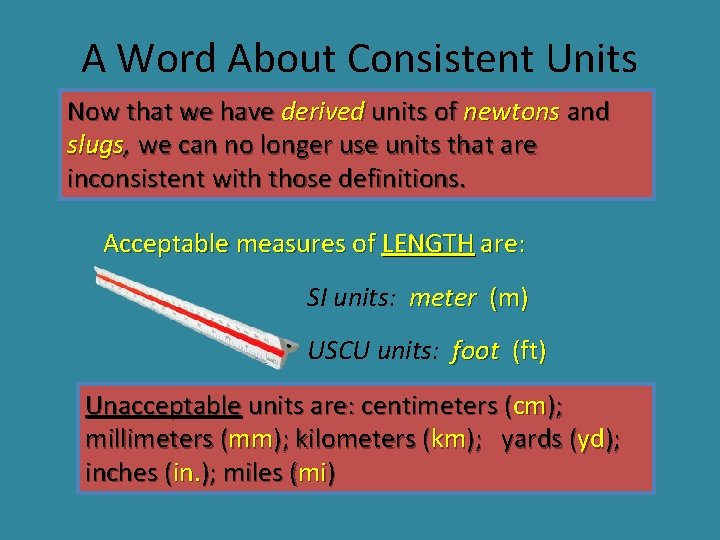A Word About Consistent Units Now that we have derived units of newtons and slugs, we can no longer use units that are inconsistent with those definitions. Acceptable measures of LENGTH are: SI units: meter (m) USCU units: foot (ft) Unacceptable units are: centimeters (cm); millimeters (mm); kilometers (km); yards (yd); inches (in. ); miles (mi)Consistent Units (Continued. . . ) Acceptable measures of MASS are: SI units: kilogram (kg) USCU units: slug (slug) Unacceptable units are: grams (gm); milligrams (mg); newtons (N); pounds (lb); ounces (oz) The last three unacceptable units are actually units of force instead of mass.Consistent Units (Continued. . . ) Acceptable measures of FORCE are: SI units: newton (N) USCU units: pound (lb) Unacceptable units are: kilonewtons (k. N); tons (tons); ounces (oz); kilograms (kg); slugs (slug) The last two unacceptable units are not force units—they are units for mass.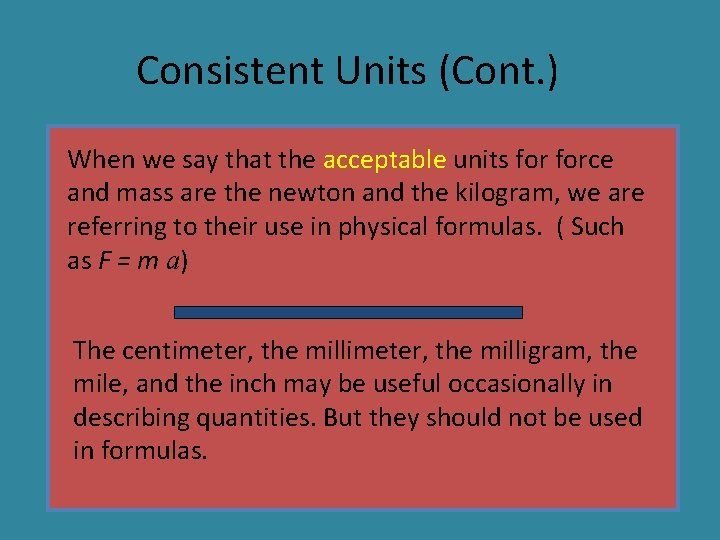Consistent Units (Cont. ) When we say that the acceptable units force and mass are the newton and the kilogram, we are referring to their use in physical formulas. ( Such as F = m a) The centimeter, the milligram, the mile, and the inch may be useful occasionally in describing quantities. But they should not be used in formulas.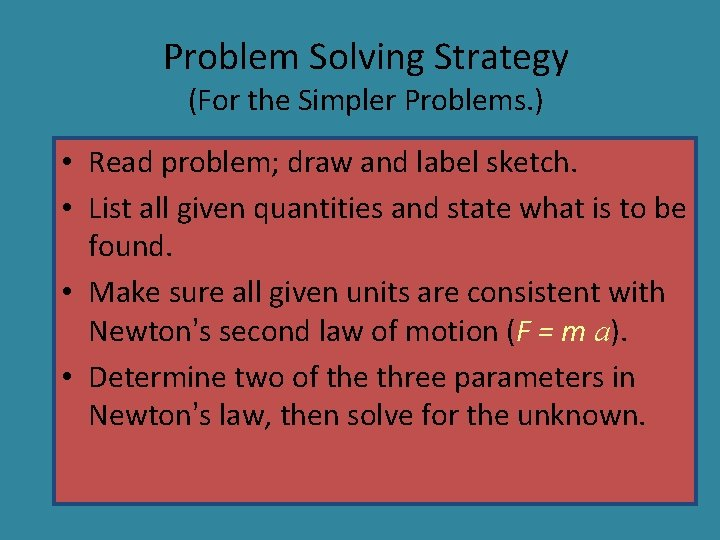Problem Solving Strategy (For the Simpler Problems. ) • Read problem; draw and label sketch. • List all given quantities and state what is to be found. • Make sure all given units are consistent with Newton’s second law of motion (F = m a). • Determine two of the three parameters in Newton’s law, then solve for the unknown.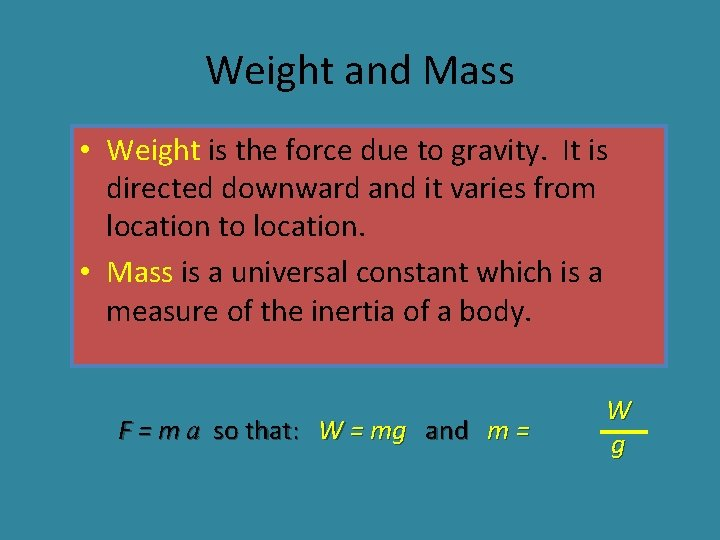Weight and Mass • Weight is the force due to gravity. It is directed downward and it varies from location to location. • Mass is a universal constant which is a measure of the inertia of a body. F = m a so that: W = mg and m = W g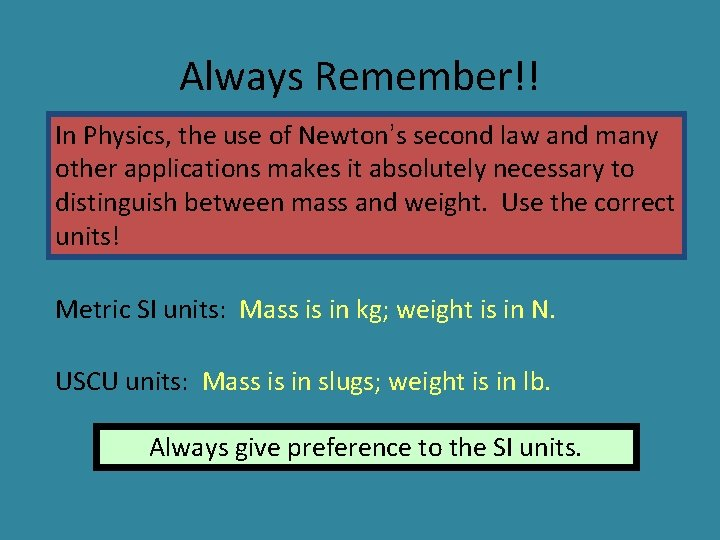Always Remember!! In Physics, the use of Newton’s second law and many other applications makes it absolutely necessary to distinguish between mass and weight. Use the correct units! Metric SI units: Mass is in kg; weight is in N. USCU units: Mass is in slugs; weight is in lb. Always give preference to the SI units.Newton’s Third Law (Reviewed): • Third Law: For every action force, there must be an equal and opposite reaction force. Forces occur in pairs. Action Reaction ActionActing and Reacting Forces • Use the words by and on to study action/reaction forces below as they relate to the hand the bar: Action The action force is exerted by the _____ on the _____. bar hands The reaction force is exerted by the _____ on the _____. bar Reaction hands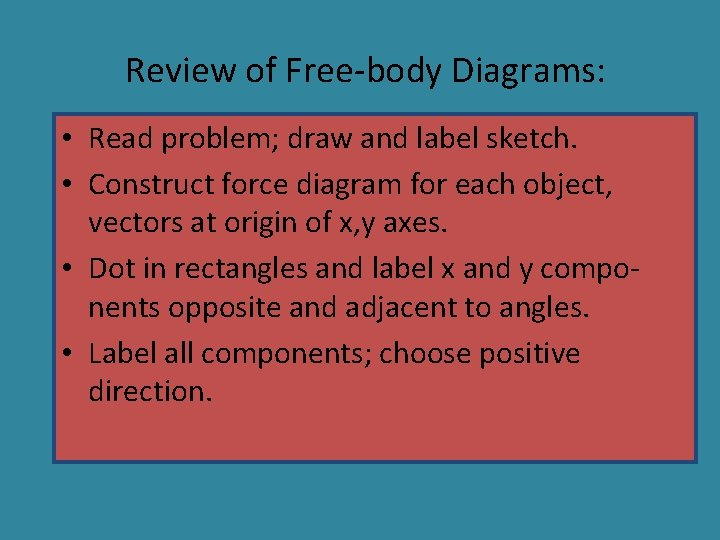Review of Free-body Diagrams: • Read problem; draw and label sketch. • Construct force diagram for each object, vectors at origin of x, y axes. • Dot in rectangles and label x and y components opposite and adjacent to angles. • Label all components; choose positive direction.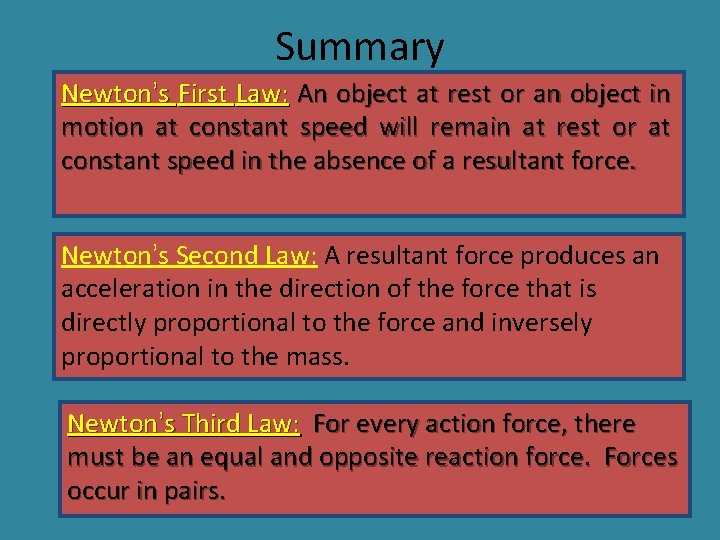Summary Newton’s First Law: An object at rest or an object in motion at constant speed will remain at rest or at constant speed in the absence of a resultant force. Newton’s Second Law: A resultant force produces an acceleration in the direction of the force that is directly proportional to the force and inversely proportional to the mass. Newton’s Third Law: For every action force, there must be an equal and opposite reaction force. Forces occur in pairs.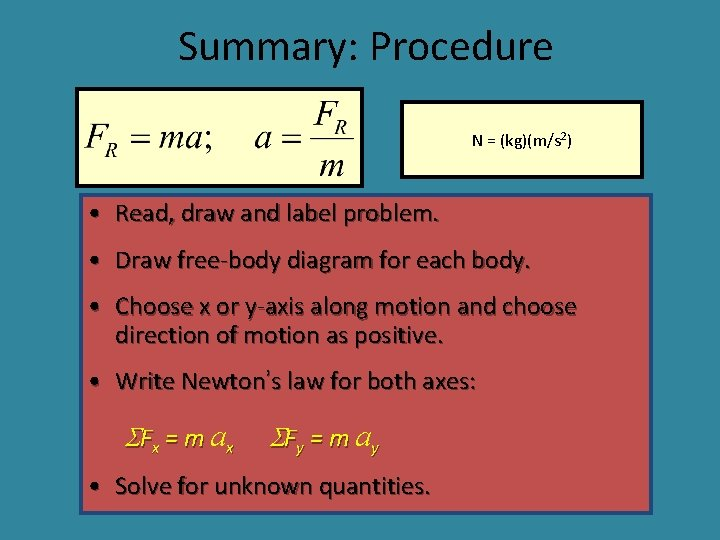Summary: Procedure N = (kg)(m/s 2) • Read, draw and label problem. • Draw free-body diagram for each body. • Choose x or y-axis along motion and choose direction of motion as positive. • Write Newton’s law for both axes: SFx = m ax SFy = m ay • Solve for unknown quantities.